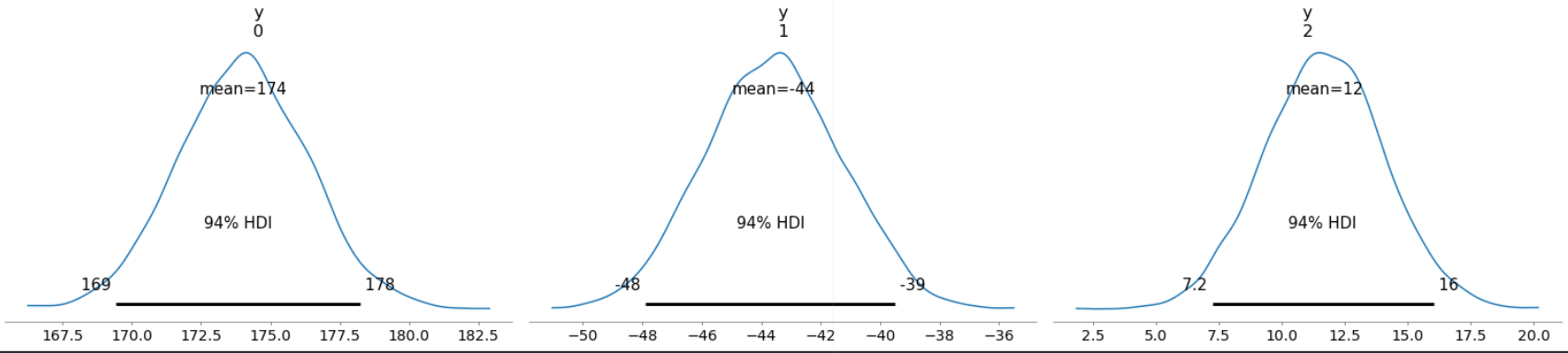# Bayesian Modeling with PYMC3

#### 04/27/2021

# load python
library(reticulate)
use_python('C:/Users/Andrew/Anaconda3/')
use_condaenv(condaenv='mcmc', required=TRUE)
library(knitr)
# load packages
import matplotlib.pyplot as plt
import numpy as np
import pandas as pd
import pymc3 as pm
## WARNING (theano.configdefaults): g++ not available, if using conda: conda install m2w64-toolchain
## C:\Users\Andrew\ANACON~1\envs\mcmc\lib\site-packages\theano\configdefaults.py:697: UserWarning: DeprecationWarning: there is no c++ compiler.This is deprecated and with Theano 0.11 a c++ compiler will be mandatory
##   "DeprecationWarning: there is no c++ compiler."
## WARNING (theano.configdefaults): g++ not detected ! Theano will be unable to execute optimized C-implementations (for both CPU and GPU) and will default to Python implementations. Performance will be severely degraded. To remove this warning, set Theano flags cxx to an empty string.
## WARNING (theano.tensor.blas): Using NumPy C-API based implementation for BLAS functions.
import arviz as az
import theano
import xarray as xr
from sklearn.datasets import make_regression
np.random.seed(1) # set seed

# 1 Introduction

Bayesian models begin with one set of plausibilities assigned to each parameter; called prior plausibilities. These priors are then updated, as the model learns from the data, to produce posterior plausibilities. At each step as the model learns, the updated set of plausibilities becomes the new initial plausibilities for the next observation. In other words, the Bayesian model updates the prior distributions with their logical consequences: the posterior distribution. This is because, for every unique combination of data, likelihood, parameters, and prior, there is a unique posterior distribution. This distribution contains the relative plausibility of different parameter values, conditional on the data and model. The posterior distribution contains the probability of the parameters given the data. The posterior is derived by:

$\text{Posterior} = \frac{\text{Probability of the data} \times \text{Prior}}{{\text{Average probability of the data}}}$

The key takeaway is that the posterior is proportional to the product of the prior and the probability of the data.

## 1.1 Key Terms:

Likelihood – relative number of ways that a parameter can produce the data; but more commonly understood as the distribution function Prior Probability – the prior plausibility for any specific parameter Posterior Probability – the updated plausibility for any specific parameter

# 2 Markov Chain Monte Carlo

The goal of MCMC is to sample from f(x) or to approximate the expected value of f[h(X)]. MCMC is comprised of two processes: Monte Carlo and Markov Chains. Monte Carlo refers to the process of simulating data while Markov Chains approximate a target probability distribution in that where we go next to sample depends only on our last state. MCMC works well because when sampling, the region of high probability tends to be connected without having to sample through low-probability regions. MCMC also has a process called burn in. Burn in reflects the time the chain takes to converge to its stationary distribution. The samples drawn from this period are expected to not closely approximate the function and therefore we remove these samples before computing the expectation of the function. When looking at MCMC chains, we look for convergence. If the trace plot shows no trends but instead shows random variability in sampling portions of the parameter space, then we say that the chain has converged. One way to check these plots are to look at autocorrelation plots as they should show a slow decay. In drawing samples from the posterior, MCMC returns a large collection of parameter values and the frequencies of these values correspond to the posterior plausibilities. A picture of the posterior is obtained by generating a histogram of these samples.

# 3 Bayesian Data Analysis Advantages

Using Bayesian methods is advantageous for the following reasons: 1. Model Design – We can draw samples from the posterior and the prior. By reviewing what the model expects before the data is applied, it provides an intuitive way to understand the implications of our choices for the prior. 2. Model Checking – After a model learns from the data, we can simulate the implied observations to check whether or not we fit our observed $$y$$. All Bayesian models are generative. 3. Forecasting – Our parameters can be used to simulate new predictions for new cases and new future observations. These forecasts are useful in terms of applied prediction but also model criticism and evaluation.

# 4 Linear Model Example

In this section, we will specify a linear model in pymc3, using all of its latest capabilities, so as to provide a complete and state-of-the-art example.

## 4.1 Generate Data

We begin by generating some data with sklearn:

features, target, true_coef = make_regression(n_samples=100,
bias=1.0,
n_informative=3,
n_features=3,
noise=2.5,
random_state=1,
coef=True)

## 4.2 Create Coordinates

Coordinates in pymc3 refer to actual values that reflect dimensions of our data set. To use coordinates to our advantage, we generally want to set at least two: (1) denoting the length of our data set, and (2) the names of our features. We can set more, for example, by splitting our features dimension into sub-dimensions, say, treatment and covariates (see example below). The reason why we may want to be more specific here is that in the event of performing high-dimensional analysis, we may want to just interogate our treatment variable of interest and this process will let us perform additional calculations and the like more easily later.

# coords
coords = {
# dim 1: len of df
'obs_id': np.arange(len(target)),
# dim 2: feature cats.
'features': ['treatment', 'cov1', 'cov2']
# alternative spec
#'treatment': ['treatment'],
#'covariates': ['cov1', 'cov2']
}

## 4.3 Build a Bayesian Model

The next step is to build our model. It is a long line of code but it includes several features that are simply unavailable to frequentists: (1) prior predictive checks, (2) posterior predictive checks, and (3) MCMC samples of the posterior and predictive posterior.

# specify model
with pm.Model(coords=coords) as sk_lm:
'''
Bayesian Linear Model
'''
# data
feature_data = pm.Data('feature_data', features, dims=('obs_id', 'features'))

# priors
alpha = pm.Normal('alpha', mu=0, sigma=1)
betas = pm.Normal('betas', mu=[40, 90, 50], sigma=5, dims='features')

# model error
sigma = pm.Exponential("sigma", lam=1)

# matrix-dot products
m1 = pm.math.matrix_dot(feature_data, betas)

# expected value of y
mu = pm.Deterministic("mu", alpha + m1, dims='obs_id')

# Likelihood: Normal
y = pm.Normal("y",
mu=mu,
sigma=sigma,
observed=target,
dims='obs_id')

# set sampler
step = pm.NUTS([alpha, betas, sigma], target_accept=0.9)

# Inference button (TM)!
lm_trace = pm.sample(draws=1000,
step=step,
cores=4,
tune=500,  # burn in
return_inferencedata=False)

# prior analysis
prior_pc = pm.sample_prior_predictive()

# posterior predictive
ppc = pm.fast_sample_posterior_predictive(trace=lm_trace,
random_seed=1,
)
# generate inference data
lm_idata = az.from_pymc3(
trace=lm_trace,
prior=prior_pc,
posterior_predictive=ppc,
)

There are several objects in the above code that warrant further discussion:

• feature_data - A pm.Data object that refers back to our numpy array of our features. Notice that we specify its dimensions of shape (obs_id, features) which translates to (100, 3), the size of our data.

• alpha - A prior for the intercept. By setting $$\mu$$ to 0, we judge that its value is just as likely to be negative as it is to be positive.

• betas - A prior for each $$\beta$$ parameter. Note that it contains three different priors for each $$\beta$$ with a large sigma, denoting high uncertainty. If we set mu=0, it would set the same prior for all of our $$\beta$$ parameters. The reason that we have three $$\beta$$ parameters specified is because we have specified its dims argument to refer back to features located in our coords which is length 3. sigma is the standard deviation and we interpret the instantiation of this prior as: The prior for $$\mu$$ is a Gaussian prior, centered on 40 with 95% probability between 40 +/- 10. We understand the prior this way because 95% of all values are located within 2 standard deviations while 1 standard deviation approximately encompasses 68% of all values.

• sigma - The error term. The interpretation of the Exponential family here is that it is an average deviation.

• m1 - Matrix-dot product of our feature data with our $$\beta$$ parameters.

• mu - We can specify this with or without a pm.Deterministic variable. By including a pm.Deterministic variable, we can explicitly capture the $$\hat{y}$$ for each sample; a useful statistic for machine learning, for example.

pymc3 offers exceptional model graphing functionality in that it provides a visual representation of the model and the relationship between each parameter like so:

# graph of model
pm.model_to_graphviz(sk_lm)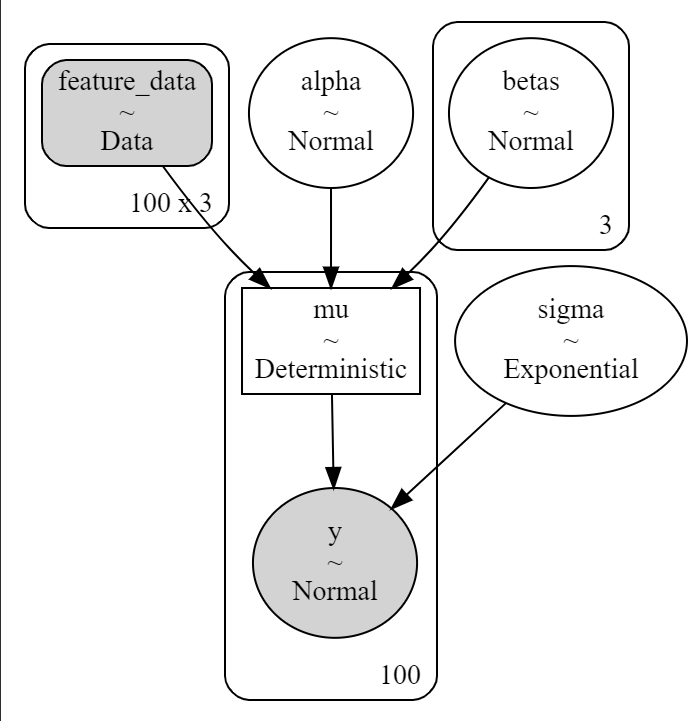## 4.4 Diagnostics: Prior Predictive Checks

Once our MCMC algorithm finishes drawing samples and discarding its burn in, we can observe several of its consequences. First, we might look at our prior predictive capacity so that we may judge the our parameter’s ability to predict $$y$$:

# diagnostics: plot prior
with sk_lm:
az.plot_ppc(data=lm_idata, num_pp_samples=100, group='prior');

The plot below draws three lines: (1) prior predictive $$y$$, (2) observed $$y$$, and (3) the prior predictive mean of $$y$$. This plot shows how close our prior choices approximate our observed data, $$y$$. In other words, the closer the black line is to the dotted blue line, the more likely our parameter choices and priors are able to predict and generate $$y$$.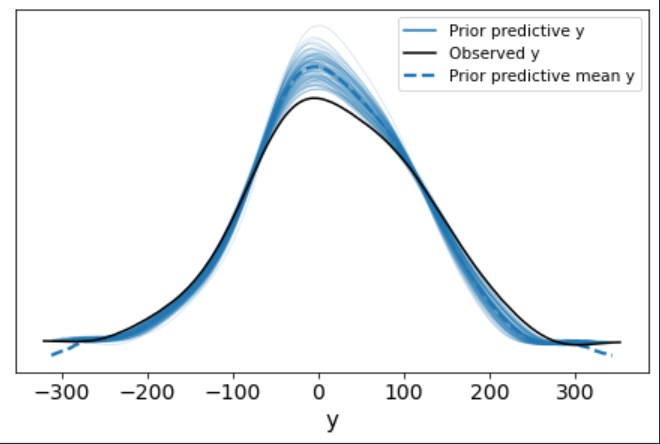## Diagnostics: Posterior Predictive Checks

The next important consequence of Bayesian modeling is our ability to conduct posterior predictive checks:

# diagnostics: plot posterior
with sk_lm:
fig, ax = plt.subplots(figsize=(12,8))
az.plot_ppc(data=lm_idata, num_pp_samples=100, group='posterior', ax=ax);
ax.axvline(np.mean(target), ls="--", color="r", label="True mean")
ax.legend(fontsize=12);

The plot below shows the extent to which our model, after learning from the data, is able to retrodict what was observed ($$y$$). A model that fits the data well is one where the black line, observed $$y$$, overlaps with the posterior predictive mean of $$y$$.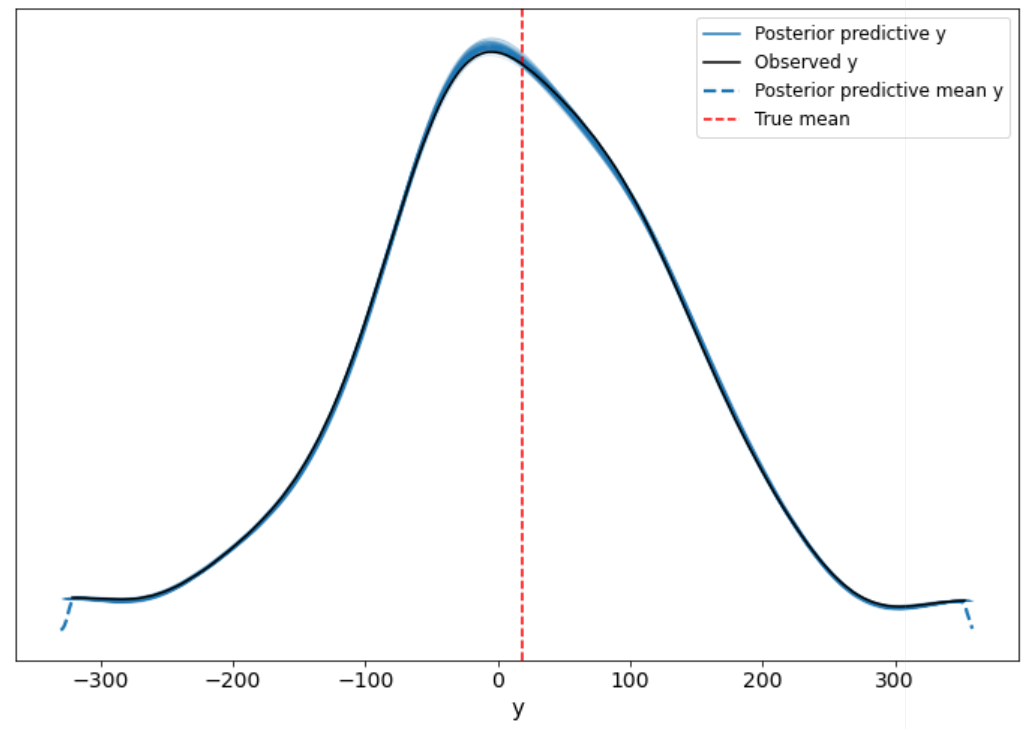## 4.5 Diagnostics: Trace Checks

Next, we can look at how our sampler fared in estimating the values of our $$\beta$$ parameter of interest for our hypothetical treatment variable:

# diagnostics: plot trace
with sk_lm:
az.plot_trace(lm_idata,
coords={ 'features': ['treatment'] },
var_names=['~mu', '~alpha', '~sigma']);

In the plot below on the left, we see the density of our parameter values across four Markov chains. Each chain is generally in agreement as the peak of each curve centers around 44.1. In the plot on the right, we see how the chain searched, with each chain sampling parameters roughly between 43.5 and 45.0. When performing visual diagnostics on chain convergence, as described above, we should observe roughly white noise, or random variabilit, which is what we see.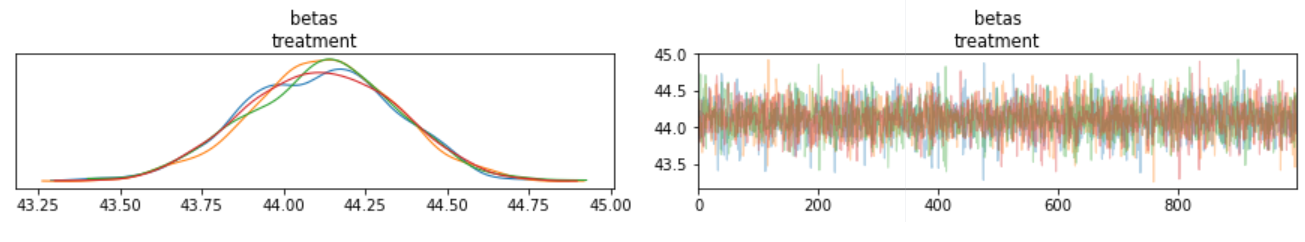Next, we evaluate our trace diagnostics in a different way be evaluating its r-hat; a diagnostic that attempts to flag situations where the MCMC algorithm failed to converge. The basic idea is that you want to check a couple of things:

1. Is the distribution of the first part of a chain (after warm up) the same as the distribution of the second half of the chain?
2. If I start the algorithm at two different places and let the chain warm up, do both chains have the same distribution?
# diagnotics: plot r-hat
az.summary(lm_idata, kind='diagnostics', var_names=['~mu'])

## 4.6 Results: 94% High Density Interval

Intervals of posterior probability are called Confidence Intervals in Bayesian terms. The interval tells us the range of parameter values compatible with the model and data.

with sk_lm:
az.plot_posterior(lm_idata,
coords={ 'features': ['treatment'] },
var_names=['~mu', '~alpha', '~sigma']);

The graph below tells us:

94% of the posterior probability lies between 44 and 45. This means that parameter values close to 43.9 or greatly above 45 are highly incompatible with the data and model. For the mean, and since there are many theoretical slopes, a line with a slope of 44 is the most likely one.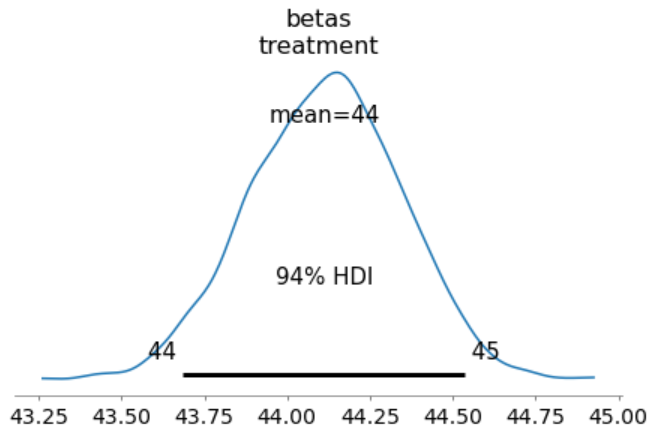## 4.7 Results: Parameter Values

pymc3 and arviz offer many more features. The code below displays our $$\beta$$ parameters of interest.

# view coefs
az.summary(lm_idata, var_names=["betas"], kind='stats')

We interpret our parameters in a Bayesian framework exactly like we would as a frequentist:

• $$\alpha$$: what is the expected outcome when $$x_{i}$$ = $$\bar{x}$$? Or $$\beta$$ = 0?

• $$\beta$$: What is the change in the expected outcome when $$x_{i}$$ changes by 1 unit; it’s the rate of change in expectation.

## 4.8 Posterior Analysis: Y-hat

To do work in the posterior, we first generate a few objections as convenience:

# grab the posterior results
post = lm_idata.posterior
# extract the data used to build the model
const = lm_idata.constant_data
• post contains our sampled parameters across four Markov chains with 1000 samples each. Within this object we have parameters for alpha, three beta parameters, sigma and mu.

• const contains our observed X data.

To get $$\hat{y}$$, we can extract it like so because we set as pm.Deterministic variable. This is convenient in the event we are aiming to do applied prediction and we want to compare our predicted values to the true values.

post['mu'].mean(dim=("chain", "draw")).values

## 4.9 Posterior Analysis: Counterfactuals

The most common quantity of interest that we are interested in generating from our posterior is a counterfactual: the change in $$y$$ for a one-unit change in $$x$$ while holding all other variables constant. To do so, we need to generate a new array with the sequence of values we are interested in estimating while also incorporating the mean of the covariates. The below code does this for us:

# Counterfactual Plot: Hold Covariates Constant:
# treatment variable column
idx_pred = 0
# indices for the covariates
idx_covs = [1, 2]
# generate low and high values to vary
low, high = np.zeros(features.shape), np.zeros(features.shape)
# vary X1 from 1 to 3
low[idx_pred], high[idx_pred] = 1, 3
# generate 25 evenly spaced observations
treatment_seq = np.linspace(start=low, stop=high, num=25)
# hold other vars at their mean; find mean
cov1_mu, cov2_mu = np.mean(features[:, 1]), np.mean(features[:, 2])
treatment_seq[:, idx_covs] = cov1_mu, cov2_mu

# compute counterfactual probabilities:
with sk_lm:
# set the new data
pm.set_data({"feature_data": treatment_seq})
# run posterior predictive sampling
post_checks = pm.fast_sample_posterior_predictive(
lm_trace)

# get y-hat
estimated_mu = post_checks['y'].mean(axis=0)

# plot
fig, ax = plt.subplots(figsize=(12,8))
ax.plot(treatment_seq[:, idx_pred], estimated_mu[:, ])
az.plot_hpd(treatment_seq[:, idx_pred], post_checks['y'][:, :], ax=ax)
ax.set_xlabel(f"Treatment")
ax.set_ylabel(f"Expected value of Y")
ax.set_title("Other predictors held at mean")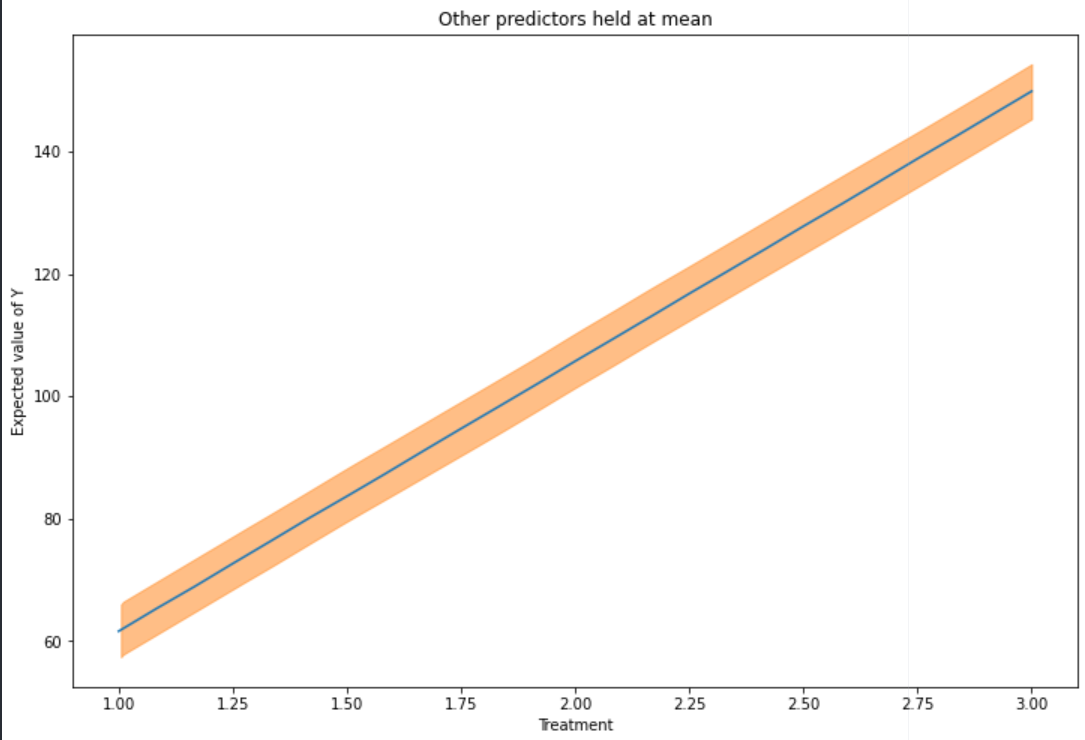## 4.10 Posterior Analysis: Out-of-Sample Data

Lastly, another common method we are interested in exploring is generating out-of-sample predictions based on new data. We conduct that analysis as follows:

# Predictions
# generate new data
new_data = np.random.randn(3, 3)

with sk_lm:
# set it over the feature data
pm.set_data({ "feature_data": new_data})
# generate preds
predictions = pm.fast_sample_posterior_predictive(lm_idata)
preds = az.from_pymc3_predictions(predictions,
coords={ 'obs_id': [0, 1, 2] },
idata_orig=lm_idata,
inplace=False)

# get y-hat for new X data
preds.predictions['y'].median(dim=('chain', 'draw')).values

# view y-hat as 94% HDI
az.plot_posterior(preds, group="predictions");